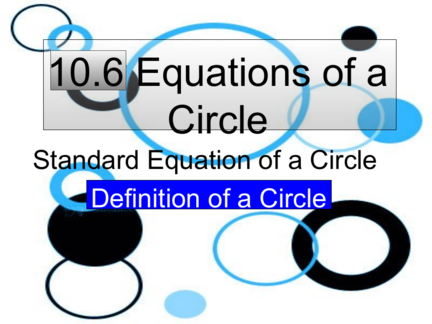# Equations of a CircleContributed by:Here in this topic, we will cover the equation of circles on a coordinate plane.
1. 10.6 Equations of a
Circle
Standard Equation of a Circle
Definition of a Circle
2. Definition of a Circle
A circle is a set of points a given distance
from one point called the center.
The distance from the center is called the
3. Standard equation of the circle
If the circle is at the origin
If the circle is not at the origin
2 2
 x  h   y  k  r 2
The center is at (h, k)
4. Standard equation of the circle
If the circle is at the origin
If the circle is not at the origin
2 2
 x  h   y  k  r 2
Solve for r
2 2
r  x  h   y  k
5. Write the equation of Circle
Center at ( -5,0) and radius 4.8
2 2
 x  h   y  k  r 2
h =?
k =?
r = 4.8
6. Write the equation of Circle
Center at ( -5,0) and radius 4.8
2 2
 x  h   y  k  r 2
h =-5 2 2 2
k =0
 x    5    y   0    4.8
r = 4.8
2
 x  5 2
 y 23.04
7. Write the equation of Circle
Center at ( 4, -3) and
a point on the circle (2,1)
2 2
 x  h   y  k  r 2
8. Write the equation of Circle
Center at ( 4, -3) and
a point on the circle (2,1)
2 2
 x  h   y  k  r 2
k=-3 2
 2  4  1    3  2
r 2
r= 2 2
x=2   2   4 r 2
y=1 4  16 r 20 2
9. Write the equation of Circle
Center at ( 4, -3) and
a point on the circle (2,1)
2 2
 x  h   y  k  r 2
k=-3 2 2
r2 = 20
 x  4   y    3  20
2 2
x=2  x  4   y  3 20
10. Given the equation of the Circle
(x – 13)2 + (y – 6)2 = 52
Tell if the point is inside or outside the circle.
(5, 6)
11. Given the equation of the Circle
(x – 13)2 + (y – 6)2 = 52
Tell if the point is inside or outside the circle.
(5, 6) (5 -13)2 + (6 – 6)2 is it <, >, or = 52
( - 8)2 + 02 = 64 = 25
Greater then outside the circle.
Less then Inside the circle.
Equal is on the circle.
12. Given the equation of the Circle
(x – 13)2 + (y – 6)2 = 52
Tell if the point is inside or outside the circle.
(5, 6) Outside
13. Given the equation of the Circle
(x – 13)2 + (y – 6)2 = 52
Tell if the point is inside or outside the circle.
(5, 6) Outside
(14,8) In
(20, 9)
14. Given the equation of the Circle
(x – 13)2 + (y – 6)2 = 52
Tell if the point is inside or outside the circle.
(5, 6) Outside
(14,8) In
(20, 9) Outside
15. Given the equation of the Circle
(x – 13)2 + (y – 6)2 = 52
Tell if the point is inside or outside the circle.
(5, 6) Outside
(14,8) In
(20, 9) Outside
(16, 2)
16. Given the equation of the Circle
(x – 13)2 + (y – 6)2 = 52
Tell if the point is inside or outside the circle.
(5, 6) Outside
(14,8) In
(20, 9) Outside
(16, 2) On
17. Homework
Page 638 – 639
# 7 – 25 , 33 - 39